Directions for questions 1 -5: Answer the following questions based on the table below, which reports certain data series from National Accounts Statistics of India at Current Prices.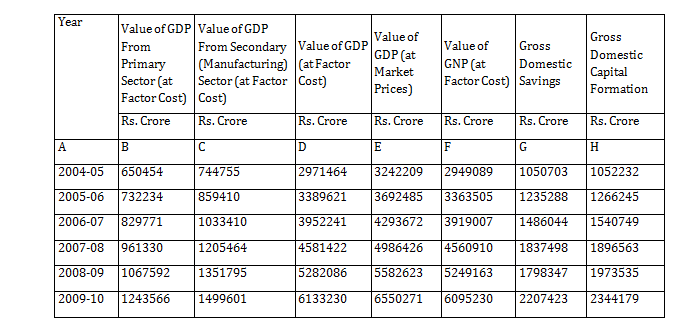Question 1. The GDP is sum total of the contributions from primary sector, secondary sector and the tertiary sector. If that be the case, then over 2004-05 to 2009-10, the share of tertiary sector at factor cost in GDP has increased from:
A. 53.05 percent to 55.27 percent
B. 52.86 percent to 54.20 percent
C. 53.04 percent to 55.83 percent
D. 52.70 percent to 56.14 percent

Question 2. The annual growth rate in the GNP series at factor cost was highest between;
A. 2008-09 and 2009-10
B. 2006-07 and 2007-08
C. 2007-08 and 2008-09
D. 2005-06 and 2006-07

Question 3. Had Gross Domestic Savings (CDS) between 2008-09 and 2009-10 increased by 30 percent, then during 2009-10 CDS expressed as a percentage of GDP at market prices would have been:
A. 33.70 percent
B. 36.85 percent
C. 35.69 percent
D. None of the above

Question 4. Mark the highest figure from the following:
A. Percentage change in GDP from Secondary sector (at Factor Cost) between 2006-07 and 2007-08.
B. Percentage change in GDP at Market Prices between 2008-09 and 2009-10.
C. Percentage change in Gross Domestic Savings between 2004-05 and 2005-06.
D. Percentage change in Gross Domestic Capital Formation between 2008-09 and 2009-10.

Question 5. Identify the correct Statement:
A. GDP (at Factor Cost) expressed as a percentage of GNP (at Factor Cost) has increased consistently between 2004-05 and 2009-10.
B. GDP (at Factor Cost) expressed as a percentage of GDP (at Market Prices) has increased, consistently between 2004-05 and 2008-09.
C. Gross Domestic Capital Formation expressed as a percentage of GDP (at Market Prices) has increased consistently between 2004-05 and 2007-08.
D. Contribution to GDP from Primary Sector (at Factor Cost) expressed as a percentage of GDP (at Factor Cost) has decreased consistently between 2004-05 and 2009-10.

Value (in Rs. crore) of tertiary sector in 2004-05

= 2971464 – (650454 + 744755)

= 1576255

Share of tertiary sector in 2004-05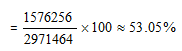Value (in Rs. crore) of tertiary sector in 2009-10

= 6133230 – (1243566 + 1499601)

= 3390063

Share of tertiary sector in 2009-10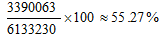Growth rate in GNP series between :

2005-06 and 2006-07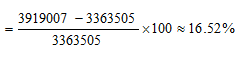2006-07 and 2007-08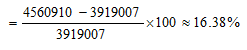2007-08 and 2008-09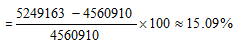2008-09 and 2009 – 10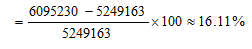Therefore, it is highest between 2005 – 06 and 2006 – 07.

Value (in Rs. crore) of GDS in 2009-10

= 1.3 × 1798347 = 2337851

Required percentage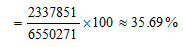Percentage change in Gross Domestic Capital Formation between 2008 – 09 and 2009 -10 is the highest and is equal to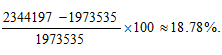Gross Domestic Capital Formation as percentage of GDP (at Market Prices) in :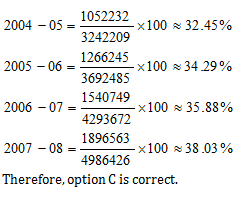­

### Want to explore more Data Interpretation Sets?

Get Posts Like This Sent to your Email
Updates for Free Live sessions and offers are sent on mail. Don't worry: we do not send too many emails..:)
Get Posts Like This Sent to your Email
Updates for Free Live sessions and offers are sent on mail. Don't worry: we do not send too many emails..:)